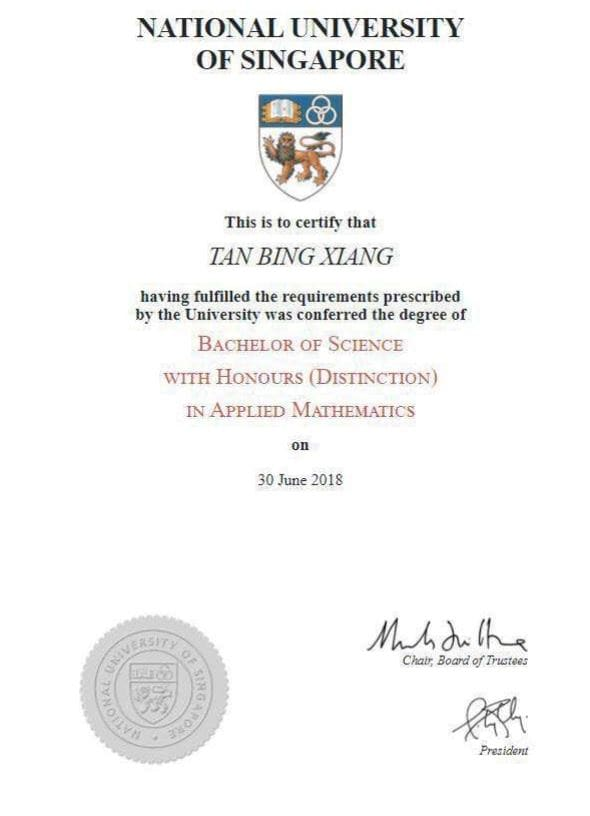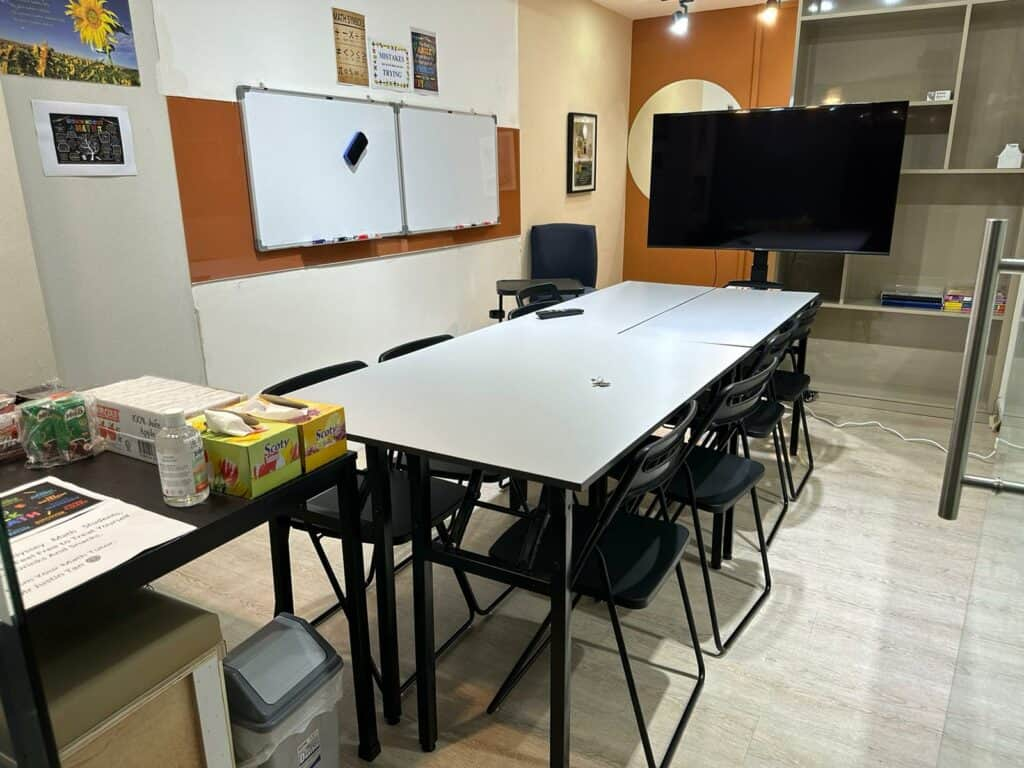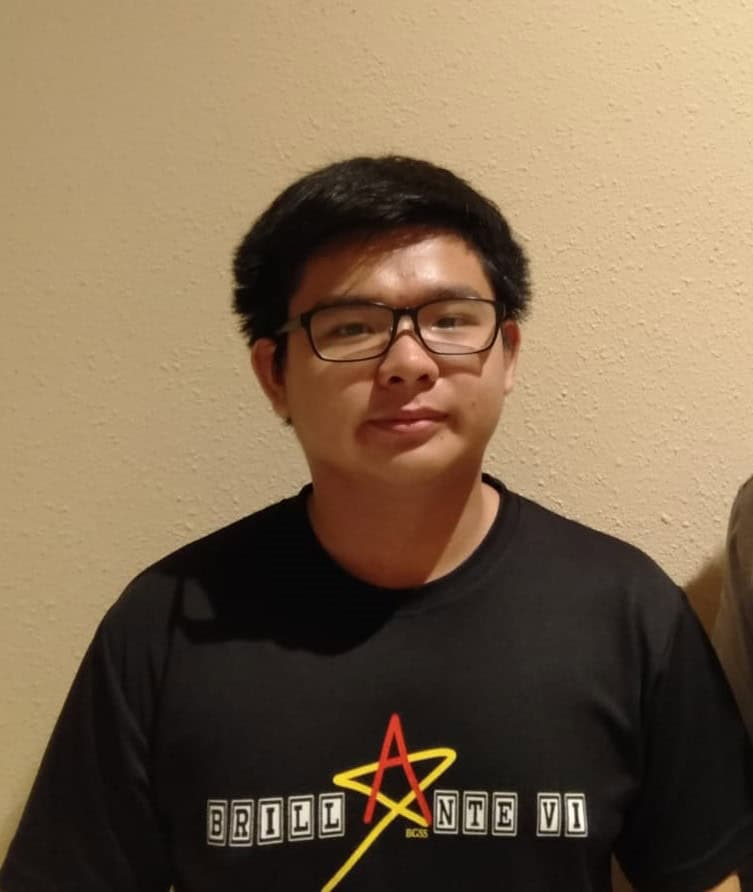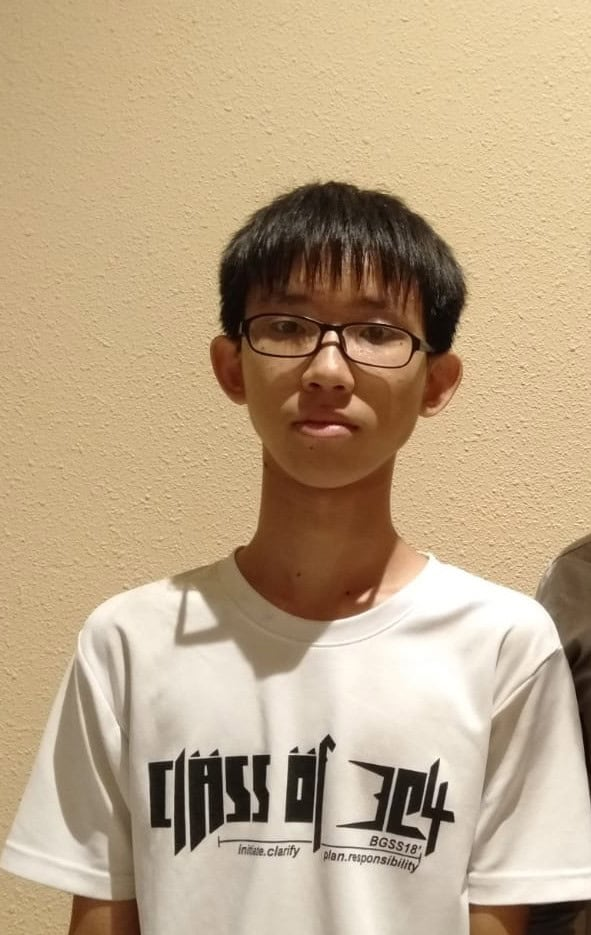# O Level E Math Tuition(Improve 2 Grades Or More)(Express/IP/NA)

## Are you worried that your child is not doing well in E Math?

E Math Tuition, how important is it? E Math requires consistency, especially when it is the culmination of math concepts learnt since secondary 1. Students struggle when they did not brush up their concepts in lower secondary days, causing them to do badly for exams. As such, students realised that they are unable to catch up at times and their bad grades roll over to the next major exams. In Odyssey Math, we are dedicated towards providing the best math resources for our students, ranging from physical notes and particularly providing them complimentary online math videos for them to recap their E Math concepts.

### \$240/ 4 weeks (Sec)\$280/ 4 weeks (JC)

Affordable, no hidden charges, no registration and administrative fees.

### Free Trial Available

First lesson is a free trial! No obligations to continue!

### Free Math Videos, over 800+

Free online math videos (see below!) for our enrolled students, totally free.

### Math Tuition

Small class size of 6 to 8, no such thing as large classes.

We ensure that even after tuition, students still get their questions answered.

## Math tuition that provides FREE e-learning for enrolled students

0 +
Hours of Development, Specially Curated For Our Math Students
0
Topical Videos and Practice Questions For Additional Math
0
Topical Videos and Practice Questions For Elementary Math
0
Topical Videos For H2 Math

## Why Odyssey Math?

### Results Oriented

The aim of coming to math tuition is for students improve their math grades through understanding math better. Here in Odyssey Math, with Mr Tan’s simplified explanation, systematic approach to learning as well as vast resources under his disposal (free math online videos), he aims to provide the best math lessons to his students. His approaches are results oriented. So long as students follow his guidance, make use of his resources and do the necessary hard work, doing well for mathematics is not impossible

### Experienced and Qualified Math Tutor

With 11 years of experience coaching mathematics and a distinction in math degree from NUS in 2018, he has taught students coming to him with a F9 grade in mathematics. Mr Tan’s students always tell him that the pace of lessons in school is just too fast. As such, students often time are unable to catch up and hence did badly for their math exams. He understands their frustration and hence aims to be the best math tutor for his students by providing lessons that are well paced and providing free online math videos for his students to recap. Mr Tan is generous particularly when it comes to providing quirky explanation for complicated concepts but it does work! The idea is sometimes to never explain things as it is but to provide an alternative of way of seeing math concepts. These are things that are not taught in textbook but rather came from his experiences teaching many students.### Affordable E Math Tuition with maximum value

Rising tuition costs has been a burden to parents in Singapore especially when they want their children to do well. Mr Tan understands this well, since he comes from a humble family where he once had to go for group tuition and the fees were pretty expensive. Now, he aims to provide affordable math tuition and at the same time give the best dedicated support to his students.

If students have math queries after lesson hours, Mr Tan always encourage them to ask even after lesson hours as he believes that learning does not stop.

### Small Class Sizes

One of the popular questions parents always ask will be how large is the class size. In Odyssey Math, we do not believe in large class size. Hence, we keep our class size small from 6 to 8 students per class.### Testimonials of our teaching

Read our testimonials from our students below to see what they have to say!

## Our E Math Tuition Structure

With learning split into 4 phases below, we will provide an introduction of math concepts to students every time we start a new chapter. Subsequently, we will answer any misconceptions they have through practice. Thereafter we will provide weekly homework assignment and review it in the subsequent lessons. Topical tests will be provided for students to test their understanding. At the end, we will do a final review and conclude the chapters and answer any leftover questions students have. When learning is split into the 4 phases below, we can better deliver the math content to students and allow them to gain mastery over the concepts.

## E Math Topics Covered

• Use of standard form
• Positive, negative, zero and fractional indices
• Laws of Indices
• Sketching the graphs of quadratic functions
• Graphs of power functions
• Graphs of exponential functions
• Estimating gradient of a curve by drawing a tangent at a point on the curve
• Sketching the graphs of quadratic functions
• Graphs of power functions
• Graphs of exponential functions
• Estimating gradient of a curve by drawing a tangent at a point on the curve
• Solving quadratic equations using formula
• Solving quadratic equations using completing the square
• Solving quadratic equations using graphical method
• Solving fractional equations by reducing it to quadratic equations
• Solving linear inequalities in one variable (inclusive of simultaneous inequalities) and subsequently represent the solution on the number line
• Formulating a quadratic equation in one variable to solve problems
• Union of A and B
• Intersection of A and B
• Number of elements in a set
• Use of notation “is an element of”
• Use of notation “is not an element of”
• Use of notation “Complement of set A”
•  Complement of set A
• The empty set
• Universal set
• A is a subset of B
• A is not a subset of B
• A is a proper subset of B
• A is not a proper subset of B
• Venn Diagrams
• Know how to display information in the form of a matrix of any order
• Know how to interpret data in a given matrix
• Know how to perform product of a scalar quantity and a matrix
• Addition, subtraction and multiplication of matrices
• Scale drawings
• Perpendicular bisectors of line segments
• Angle bisectors
• Congruence and Similarity
• Ratio of areas of similar plane figures
• Ratio of volumes of similar solids
• equal chords are equidistant from the centre
• the perpendicular bisector of a chord passes through the centre
• tangents from an external point are equal in length
• the line joining an external point to the centre of the circle bisects the angle between the tangents
• angle in a semicircle is a right angle
• angle between tangent and radius of a circle is a right angle
• angle at the centre is twice the angle at the circumference
• angles in the same segment are equal
• angles in opposite segments are supplementary
• extending sine and cosine to obtuse angles
• use of the formula for the area of a triangle (either non-right angle or right angle)
• use of sine rule and cosine rule for any triangle
• two and three dimensions problems including those involving angles of elevation and depression and bearings
• Arc length, sector area and area of a segment of a circle
• Use of radian measure of angle (conversion between radians and degrees)
• Find the gradient of a straight line given the coordinates of two points on it
• Find the length of a line segment given the coordinates of its end points
• Interpreting and finding the equation of a straight line graph in the form y=mx+c
• geometric problems involving the use of coordinates
• Know how to use notations in vectors
• Know how to represent a vector as a directed line segment
• Translation by a vector
• Position vectors
• Magnitude of a vector
• Use of sum and difference of two vectors to express given vectors in terms of two coplanar vectors
• Multiplication of a vector by a scalar
• Geometric problems involving the use of vectors
• quartiles and percentiles
• range, interquartile range and standard deviation
• cumulative frequency diagrams
• box-and-whisker plots
• calculation of standard deviation for a set of data (grouped and ungrouped)
• using mean and standard deviation to compare two sets of data
• Probability of simple combined events (either possibility diagram or tree diagram)
• Addition and multiplication of probabilities (mutually exclusive events and independent events)

## What Our Students Have to Say about Odyssey Math Tuition

Mr Tan is very dedicated in teaching A and E Math. I am able to ask questions related to mathematics and will be able to get a reply asap. Notes and questions provided are scaled to the student's personal standards. My understanding of Additional and Elementary Math has made a lot of improvements under his teaching by being able to cope better with this subject.Vernasa
Mr Tan is a very approachable, friendly and patient tutor. His teachings are easily understandable, clear and helpful. Whenever i face problems or have difficulties, he will be there to guide me and ensure that i fully understand my doubts before continuing. The math questions he provided are unique and helpful in allowing me to understand the concepts better. His math tuition has allowed me to understand mathematics better.Beyvlen Koh
In Mr Tan's math tuition, he go through his math lessons with a lot of details. I enjoyed the way he explain questions, especially when he use several methods to explain the solutions. He will provide alternative solutions for us to understand the questions better. The questions he provided allow me to have a good grasp of the math concepts. The pace of his explanation allows me to understand better. Thank you Mr Tan!Yun Tian Yi
Mr Tan is very knowledgeable in mathematics and he is very efficient in teaching his students. Even though i only spent a few sessions with him just before my O level, i have already learnt what i needed to learn based on the syllabus. I get to clarify my doubts and misconceptions in mathematics with him and he is able to answer me quickly. I would highly recommend everyone to try out his math tuition.Algernon Quek
The math questions provided are well thought-out and it is up to the standard for average students. After practicing the questions, Mr Tan will explain every questions patiently and make sure i understand not just the solutions but also the process of tackling the questions. He is always willing to answer any inquiries. Thank you so much Mr Tan!Teo Yi Hao
Mr Tan's lessons were very productive and he will always put in the extra effort to teach us after the lesson which he is not oblige to do so. He would stay after the lessons to answer our queries and go through the math questions that we asked. His explanations are clear and simple. At the end of the explanation, he made me felt like the questions were actually quite easy to tackle. Thanks a lot Mr Tan and i appreciate your passion in teaching mathematics!Beyvlen Koh
Before going for Mr Tan's math tuition, i had a lot of doubts about the sessions. After going for his sessions, it was the best decision ever made. He is a really nice teacher who is patient in teaching. From my point of view, he teaches really well and his explanation is clear and concise. I could feel that our improvement in math grades will be his greatest achievement. He always does his best in teaching and make sure that we understand the concepts better. I would recommend students to join his lessons.